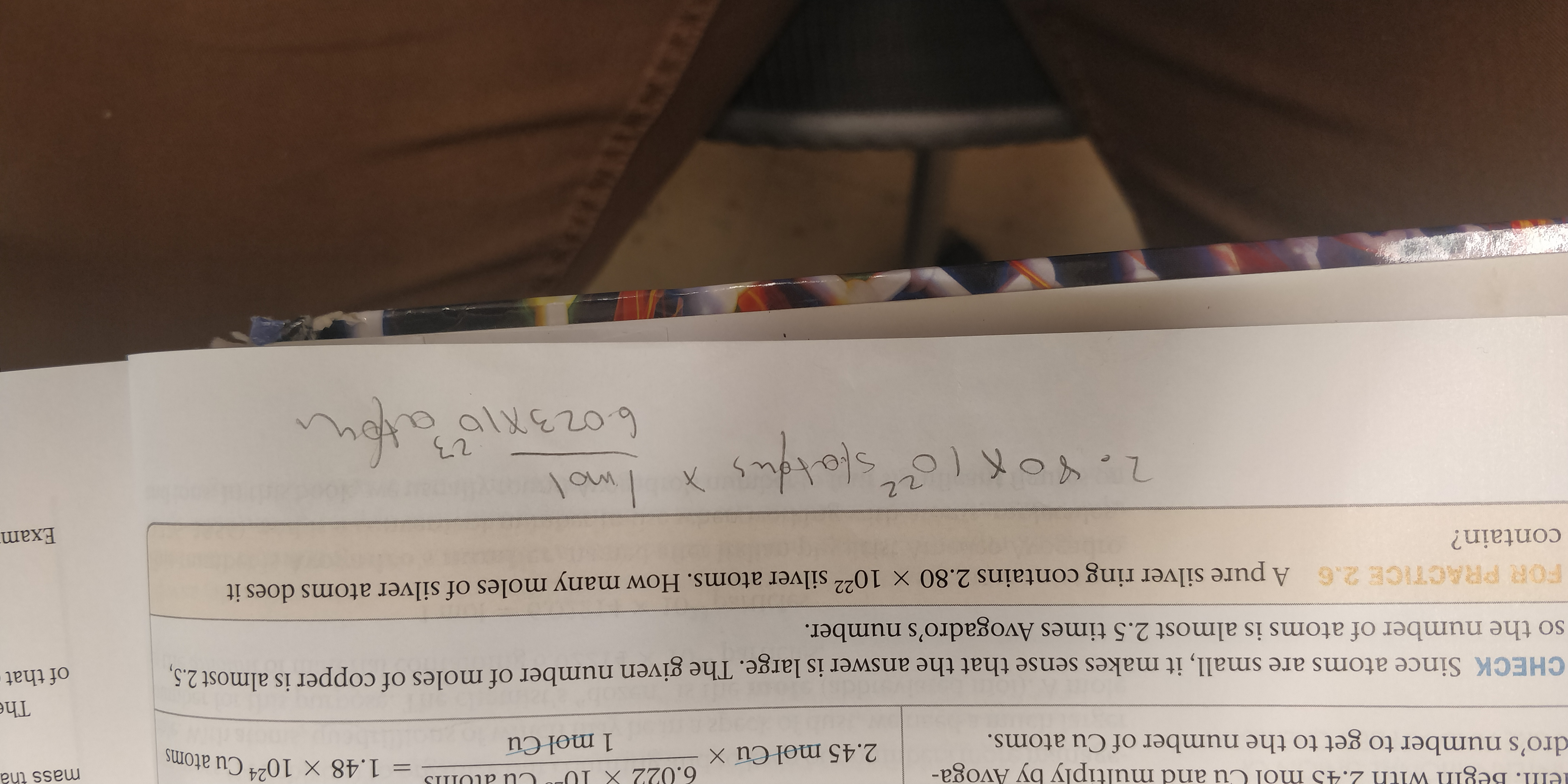ell. Begin with 2.45 mol Cu and multiply by Avoga-6.022 X 1USI P Nn= 1.48 X 10 Cu atomsmass tha242.45 mol Cu Xdro's number to get to the number of Cu atoms.1 mol CuTheCHECK Since atoms are small, it makes sense that the answer is large. The given number of moles of copper is almost 2.5,so the number of atoms is almost 2.5 times Avogadro's number.of thatFOR PRACTICE 2.6 A pure silver ring contains 2.80 x 1022 silver atoms. How many moles of silver atoms does itcontain?Exam22allezo9

Questionhelp_outlineImage Transcriptioncloseell. Begin with 2.45 mol Cu and multiply by Avoga- 6.022 X 1U SI P Nn = 1.48 X 10 Cu atoms mass tha 24 2.45 mol Cu X dro's number to get to the number of Cu atoms. 1 mol Cu The CHECK Since atoms are small, it makes sense that the answer is large. The given number of moles of copper is almost 2.5, so the number of atoms is almost 2.5 times Avogadro's number. of that FOR PRACTICE 2.6 A pure silver ring contains 2.80 x 1022 silver atoms. How many moles of silver atoms does it contain? Exam 22 allezo9 fullscreen
Step 1

Given:

Number of Silver atoms in the sample = 2.60  X 1022

We need to calculate the number of moles of Ag in the given sample.

Step 2

Calculation:

1 mole of any substance contains Avogadro number of atoms ( 6.022 X 10 23)

Now, in the given sample we have 2.60  X 1022 atoms.

If 6.022 X 10 23atoms are in 1 mole

2.60  X 1022atoms will constitute--------...

Want to see the full answer?

See Solution

Want to see this answer and more?

Our solutions are written by experts, many with advanced degrees, and available 24/7

See Solution
Tagged in

Inorganic Chemistry